PHP 数据类型

PHP 语言中有 7 种数据类类型

1 String 字符串
2 Integer 整型
3 Float 浮点型
4 Boolean 布尔型
5 Array 数组
6 Object 对象
7 NULL 空值

PHP 字符串

```<?php
\$x = "Hello world!";
echo \$x;
echo "<br>";
\$x = 'Hello world!';
echo \$x, "<br/>";

echo "<p>PHP 基础教程 - 简单教程(www.twle.cn)</p>";
```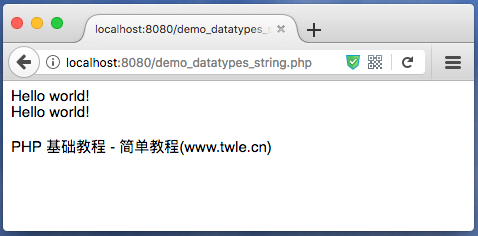PHP 整型

• 整数必须至少有一个数字 (0-9)
• 整数不能包含逗号或空格
• 整数是没有小数点的
• 整数可以是正数或负数
• 整型可以用三种格式来指定：十进制，十六进制 ( 前缀为 0x ) 或八进制 ( 前缀为 0 )

PHP `var_dump()` 函数可以输出变量的值和数据类型

范例

```<?php

\$x = 29;
var_dump(\$x);
echo "<br>";
\$x = -28; // 负数
var_dump(\$x);
echo "<br>";
\$x = 0xcc; // 十六进制数
var_dump(\$x);
echo "<br>";
\$x = 027; // 八进制数
var_dump(\$x);
echo "<p>PHP 基础教程 - 简单教程(www.twle.cn)</p>";
```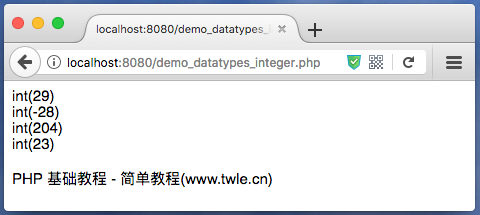PHP 浮点型

PHP `var_dump()` 函数可以输出变量的值和数据类型

范例

```<?php

\$x = 27.365;
var_dump(\$x);
echo "<br>";
\$x = 27.4e3;
var_dump(\$x);
echo "<br>";
\$x = 2E-5;
var_dump(\$x);
echo "<p>PHP 基础教程 - 简单教程(www.twle.cn)</p>";
```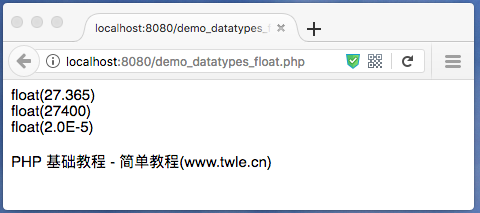PHP 布尔型

TRUE 或 FALSE 大小写都可以，不过为了好识别，一般情况下使用小写的

PHP `var_dump()` 函数可以输出变量的值和数据类型

范例

```<?php

\$x = true;
\$y = false;

var_dump(\$x);
echo "<br/>";
var_dump(\$y);

echo "<p>PHP 基础教程 - 简单教程(www.twle.cn)</p>";
```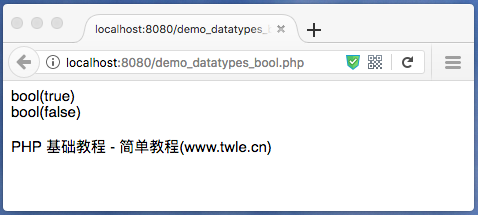PHP 数组

范例

```<?php

\$cars=array("Volvo","BMW","SAAB");
echo "<pre>";
var_dump(\$cars);
echo '</pre>';
echo "<p>PHP 基础教程 - 简单教程(www.twle.cn)</p>";
```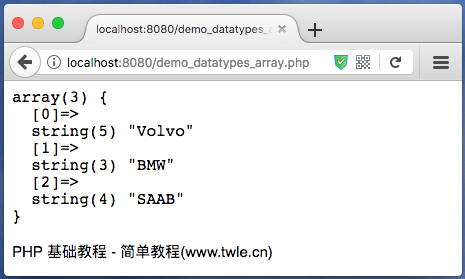PHP 对象

范例

```<?php

class Car
{
var \$color;

function __construct(\$color="green")
{
\$this->color = \$color;
}

function what_color() {
return \$this->color;
}
}

function print_vars(\$obj)
{
foreach (get_object_vars(\$obj) as \$prop => \$val)
{
echo "\t\$prop = \$val\n";
}
}

// instantiate one object
\$herbie = new Car("white");

// show herbie properties
echo "\herbie: Properties\n";
print_vars(\$herbie);

echo "<p>PHP 基础教程 - 简单教程(www.twle.cn)</p>";
```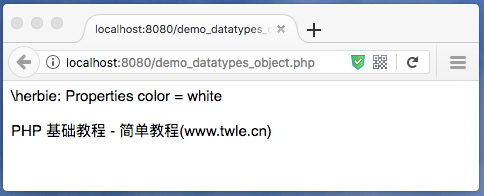PHP NULL 值

NULL 值表示变量没有值

`null` 是数据类型为 NULL 的值

`null` 不区分大小写，可以使 `null``NULL`, 但推荐使用 `NULL`;

范例

```<?php

\$x="Hello world!";
\$x=null;
var_dump(\$x);

echo "<p>PHP 基础教程 - 简单教程(www.twle.cn)</p>";
```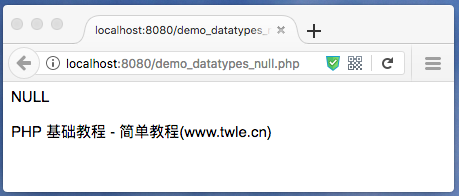PHP 基础教程

简单教程，简单编程 - IT 入门首选站